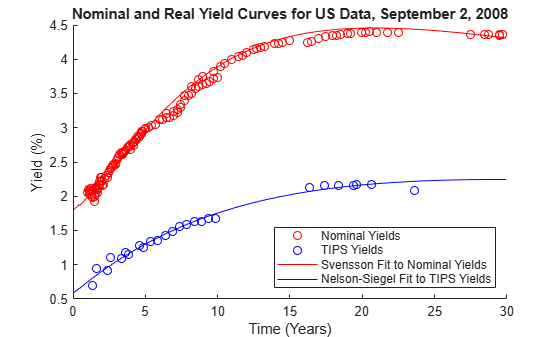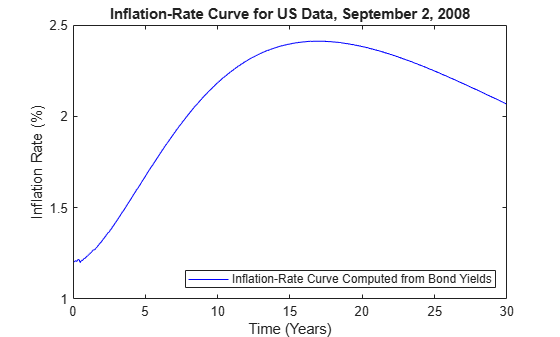# Analysis of Inflation Indexed Instruments

This example shows how to analyze inflation indexed instruments using Financial Toolbox™ and Financial Instruments Toolbox™.

### Computing Real Prices and Yields for Inflation Indexed Bonds

Inflation indexed bonds have grown in popularity and currently are issued by many countries, including the US, UK, Italy and France. While initially there was a great deal of variation in the design of inflation indexed bonds (for example, the length of the indexation lag), the majority of inflation indexed bonds now have a three month lag and are capital-indexed, that is, the principal of the bond is indexed to inflation. Therefore, the coupon rate of the bond is constant, but the actual coupon payments vary as the principal of the bond is indexed to inflation.

Specifically, the indexation is done with the following ratio

`$IndexRatio=\frac{CP{I}_{Ref}}{CP{I}_{Base}}$`

where $CP{I}_{Base}$ is the level of the Consumer Price Index (or equivalent price measure) at the time of the bond's issue, and the $CP{I}_{Ref}$ is the reference CPI.

Further, the $CP{I}_{Ref}$ is typically computed by linearly interpolating between the data two and three months previous, as in the following:

`$CP{I}_{Ref}=CP{I}_{Month-3}+\frac{SettleDay-1}{DaysInMonth}\left(CP{I}_{Month-2}-CP{I}_{Month-3}\right)$`

Computing the cash flows for an inflation indexed bond is simply a case of computing the appropriate Reference CPI and Index Ratio.

Market convention for inflation indexed bonds is to quote the price and yield using the actual (that is, unadjusted) coupon, which means that this is a real price and yield. Existing Financial Toolbox™ functions `bndprice` and `bndyield` can be used. For example:

```Price = 124 + 9/32; Settle = datenum('28-Sep-2009'); Coupon = .03375; Maturity = datenum('15-Apr-2032'); RealYield = bndyield(Price,Coupon,Settle,Maturity); disp(['Real Yield: ', num2str(RealYield*100) '%'])```
```Real Yield: 2.0278% ```

### Constructing Nominal, Real and Inflation Curves

With the advent of the inflation indexed bond market, real curves can be constructed in a similar fashion to nominal curves. Using the available market data, a real curve can be constructed which can be compared to the nominal curve.

Note that one issue relates to the indexation lag of the bonds. As discussed above, typically the indexation lag is three months, which means that the inflation compensation is not actually matched up with the maturity -- or the coupon payments -- of the bond. While Anderson and Sleath  discuss below an approach to resolving this discrepancy, for this example the lag is simply noted.

In the example below, the `IRFunctionCurve` object in the Financial Instruments Toolbox™ is used to fit Nelson Siegel and Svensson models to real and nominal yield curves in the US. Note that a typical Nelson Siegel model places restrictions on the model parameters to ensure that the interest rates are always positive. However, real interest rates can be negative, which means that the Nelson Siegel restrictions are not used in the case below.

```% Load the data load usbond_02Sep2008 Settle = datenum('02-Sep-2008'); NominalTimeToMaturity = yearfrac(Settle,NominalMaturity); TIPSTimeToMaturity = yearfrac(Settle,TIPSMaturity); % Compute the yields NominalYield = bndyield(NominalPrice,NominalCoupon,Settle,NominalMaturity); TIPSYield = bndyield(TIPSPrice,TIPSCoupon,Settle,TIPSMaturity); % Plot the yields scatter(NominalTimeToMaturity,NominalYield*100,'r') hold on scatter(TIPSTimeToMaturity,TIPSYield*100,'b') % Fit yield curves using the IRFunctionCurve object TIPSInstruments = [repmat(Settle,size(TIPSPrice,1),1) TIPSMaturity ... TIPSPrice TIPSCoupon]; TIPSSvensson = IRFunctionCurve.fitNelsonSiegel('Zero',Settle,TIPSInstruments); NominalInstruments = [repmat(Settle,size(NominalPrice,1),1) NominalMaturity ... NominalPrice NominalCoupon]; NominalSvensson = IRFunctionCurve.fitSvensson('Zero',Settle,NominalInstruments); PlotDates = Settle+30:30:Settle+365*30; PlotTimeToMaturity = yearfrac(Settle,PlotDates); plot(PlotTimeToMaturity,NominalSvensson.getParYields(PlotDates)*100,'r') plot(PlotTimeToMaturity,TIPSSvensson.getParYields(PlotDates)*100,'b') % Annotate plot title('Nominal and Real Yield Curves for US Data, September 2, 2008') xlabel('Time (Years)') ylabel('Yield (%)') legend({'Nominal Yields','TIPS Yields','Svensson Fit to Nominal Yields',... 'Nelson Siegel Fit to TIPS Yields'},'location','southeast')``````% Create an inflation curve by subtracting the real curve from the nominal % curve InflationCurve = IRDataCurve('Zero',Settle,PlotDates,... NominalSvensson.getZeroRates(PlotDates) - TIPSSvensson.getZeroRates(PlotDates));```

### Constructing Inflation Curves from Zero Coupon Inflation Swaps

Inflation linked derivatives have also experienced a great deal of growth in the last few years. Some of the most liquidly traded inflation derivatives are zero coupon inflation swaps and year on year inflation swaps.

In a zero coupon inflation swap, the inflation payer agrees to pay the rate of inflation at maturity (lagged by a certain amount) compounded by the number of years. The inflation receiver typically pays a fixed rate, again compounded by the tenor of the instrument.

Using the notation from Hurd and Relleen  below

`$\left(1+Rat{e}_{swap}{\right)}^{T}=\left(1+Inflatio{n}_{t-L,t+T-L}{\right)}^{T}$`

where t is the current time, T is the tenor and L is the lag.

Zero coupon inflation swaps can be used to build an inflation curve, which can then be compared with the inflation curve constructed from government bonds.

One point to note is that in pricing inflation derivatives and building inflation curves, incorporating seasonality can be a critical factor. This issue is not dealt with here.

In the code below, the inflation curve constructed above is plotted. It would be straightforward to add to this plot an inflation curve constructed from zero coupon inflation swaps given market data.

```figure plot(PlotTimeToMaturity,InflationCurve.getParYields(PlotDates)*100,'b') title('Inflation Curve for US Data, September 2, 2008') xlabel('Time (Years)') ylabel('Inflation (%)') legend({'Inflation Curve computed from Bond Yields'},'location','southeast')```### Bibliography

This example is based on the following papers and journal articles:

 Anderson N. and J. Sleath. "New Estimates of the UK Real and Nominal Yield Curves." Bank of England, working paper 126, 2001.

 Brigo, D. and F. Mercurio. Interest Rate Models - Theory and Practice: With Smile, Inflation and Credit. Springer, 2006.

 Deacon, M., A. Derry, and D. Mirfendereski. Inflation-Indexed Securities: Bonds, Swaps, and Other Derivatives. Wiley Finance, 2004.

 Hurd, M. and J. Relleen. "New Information from Inflation Swaps and Index-linked Bonds." Quarterly Bulletin, Spring 2006.

 Gurkaynak, R. S., and B.P. Sack, and J.H. Wright. "The TIPS Yield Curve and Inflation Compensation." FEDS Working Paper No. 2008-05, October 2008.

 Kerkhoef, J. "Inflation Derivatives Explained." Lehman Brothers.